### Search Results for: "avantika fabric"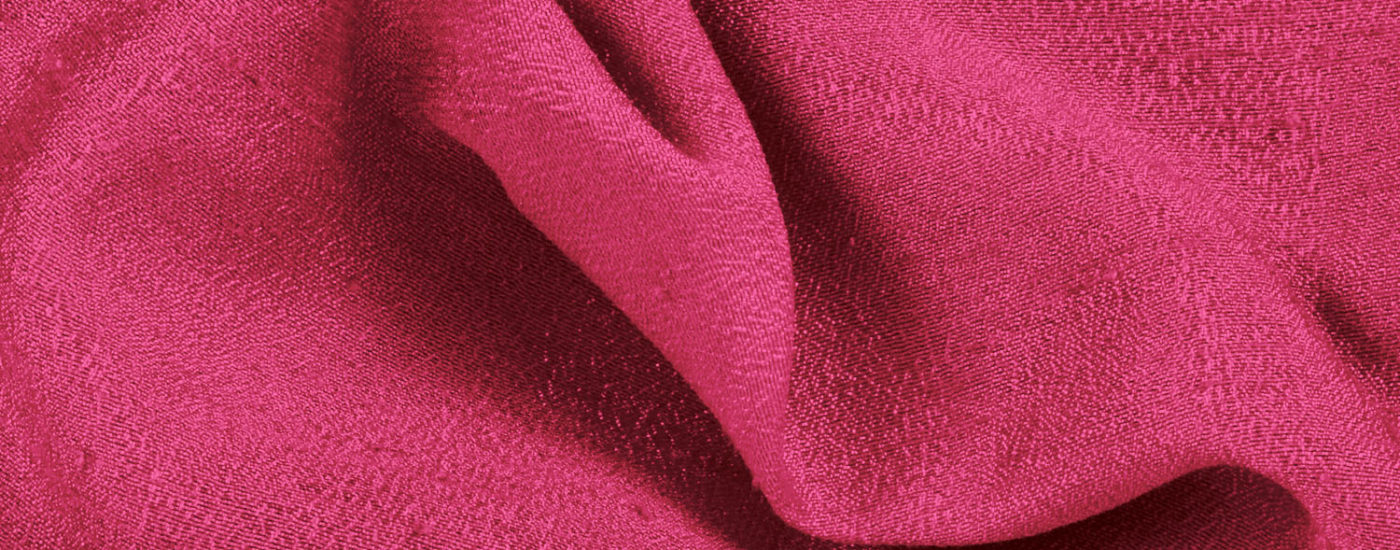Minimum Order Quantity should be 20 meters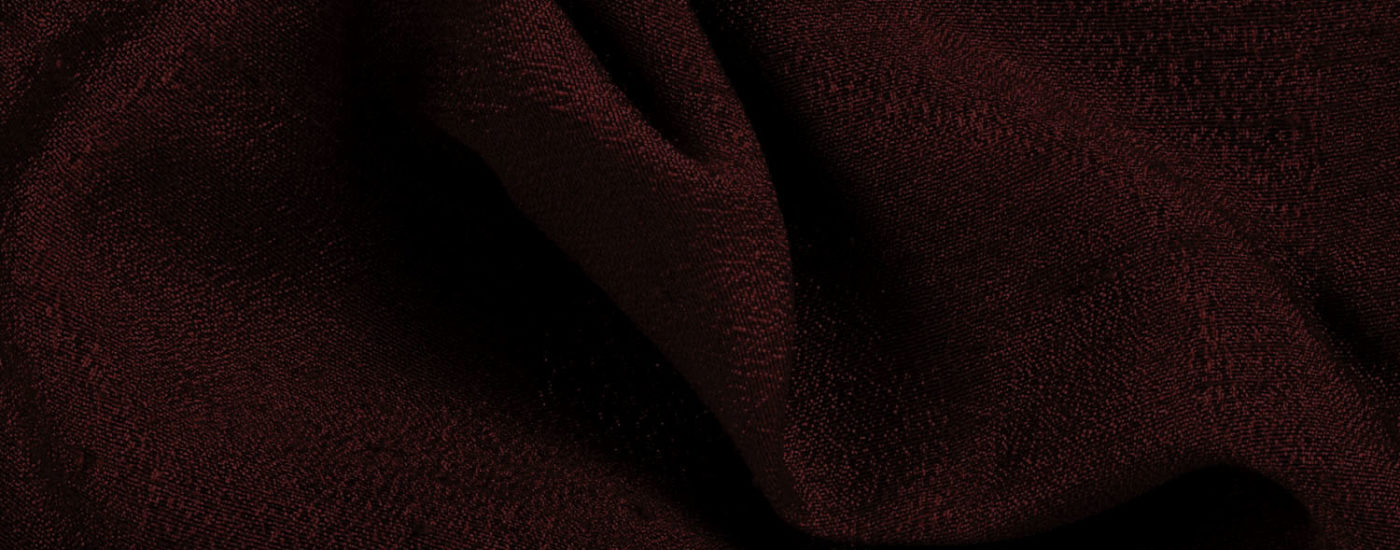Minimum Order Quantity should be 20 meters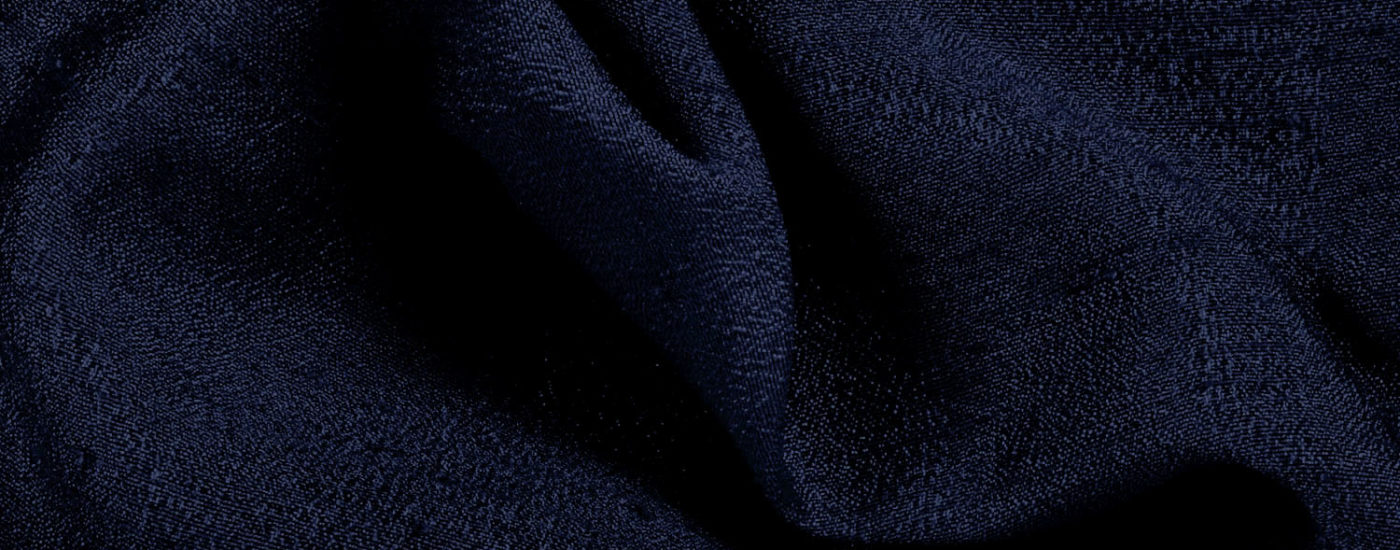Minimum Order Quantity should be 20 metersMinimum Order Quantity should be 20 meters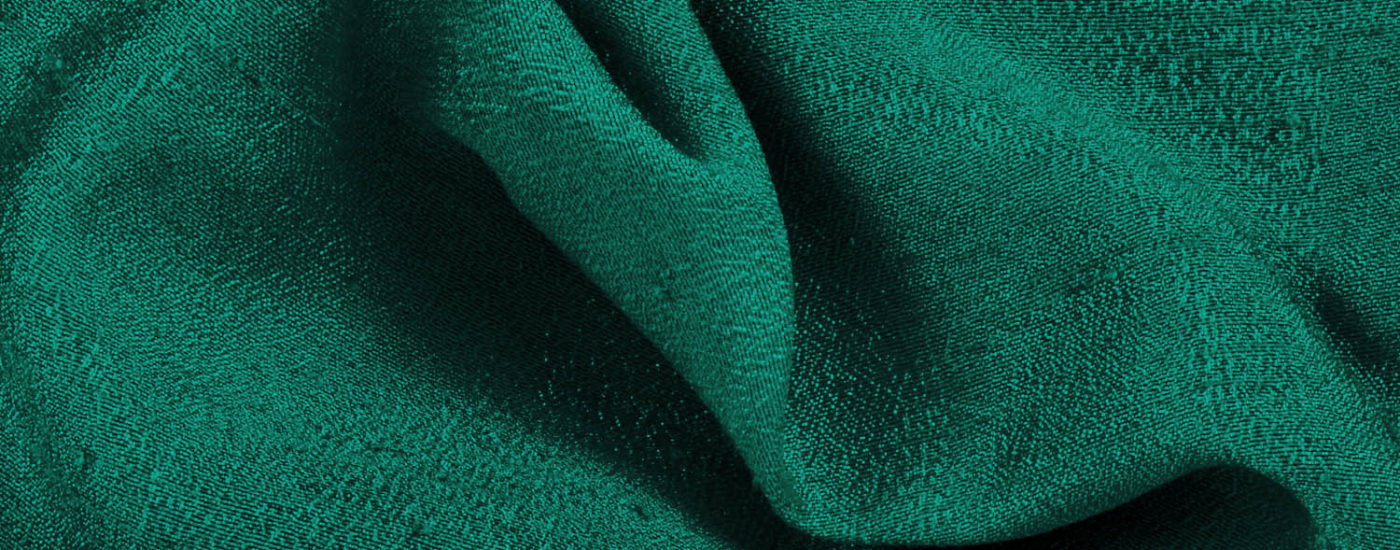Minimum Order Quantity should be 20 metersMinimum Order Quantity should be 20 meters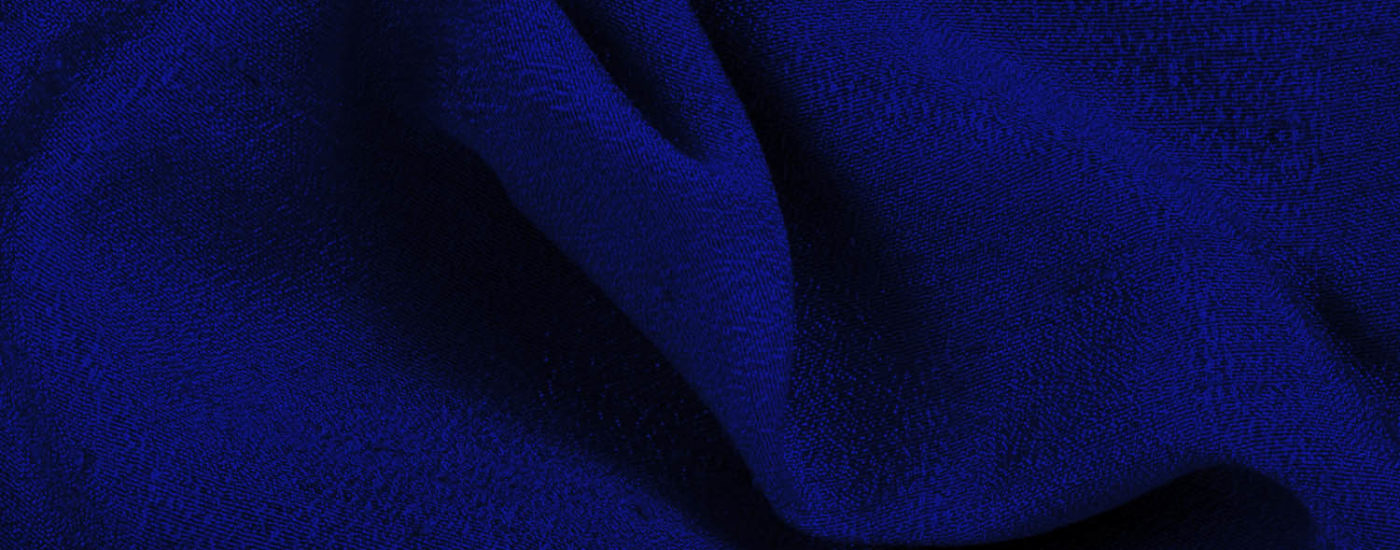Minimum Order Quantity should be 20 meters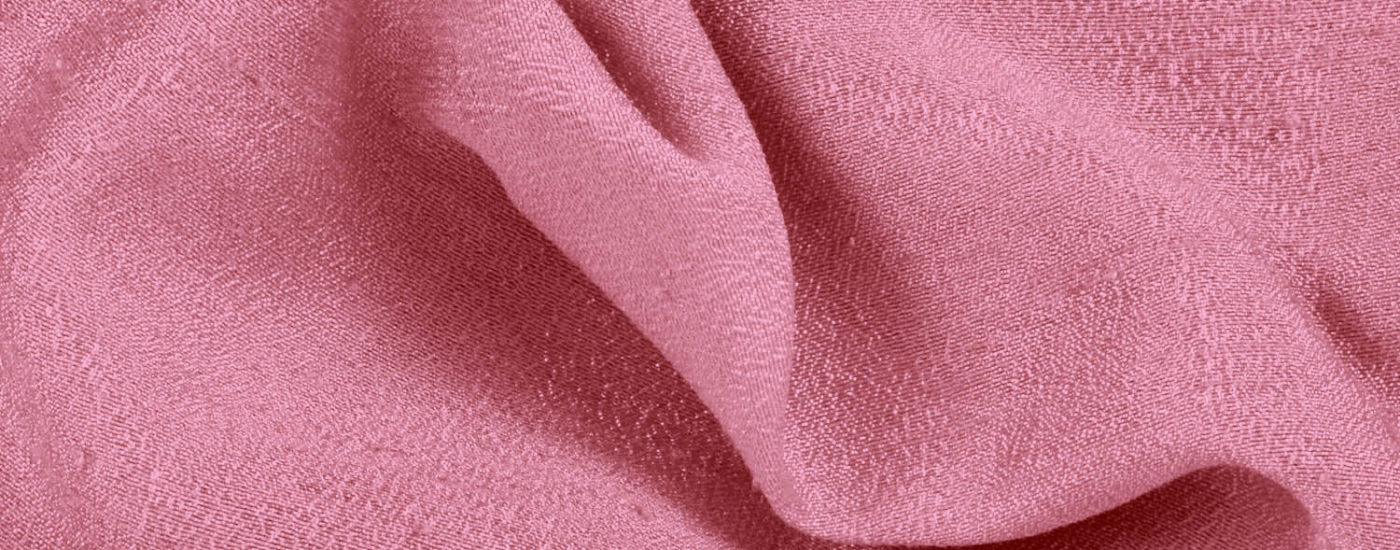Minimum Order Quantity should be 20 meters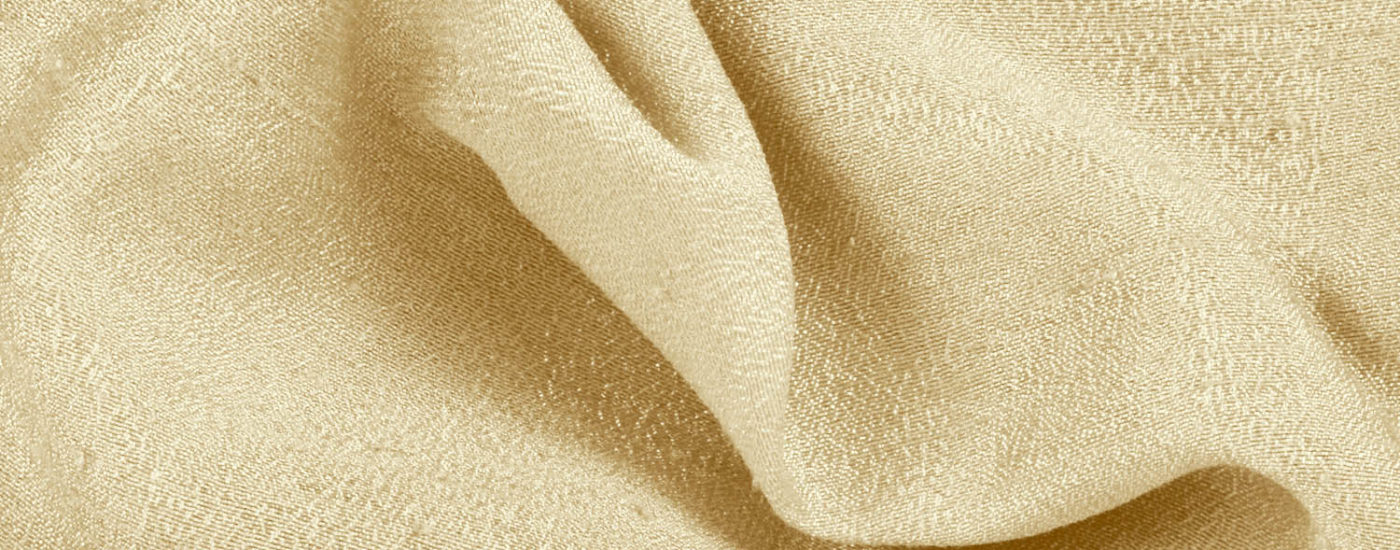Minimum Order Quantity should be 20 meters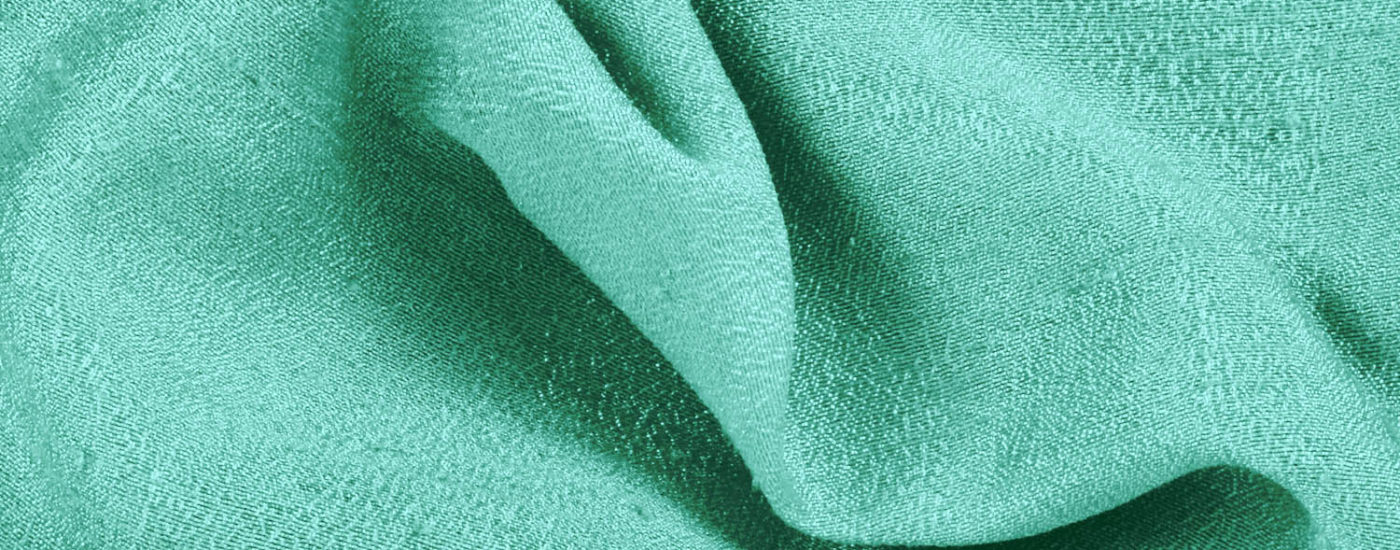Minimum Order Quantity should be 20 meters